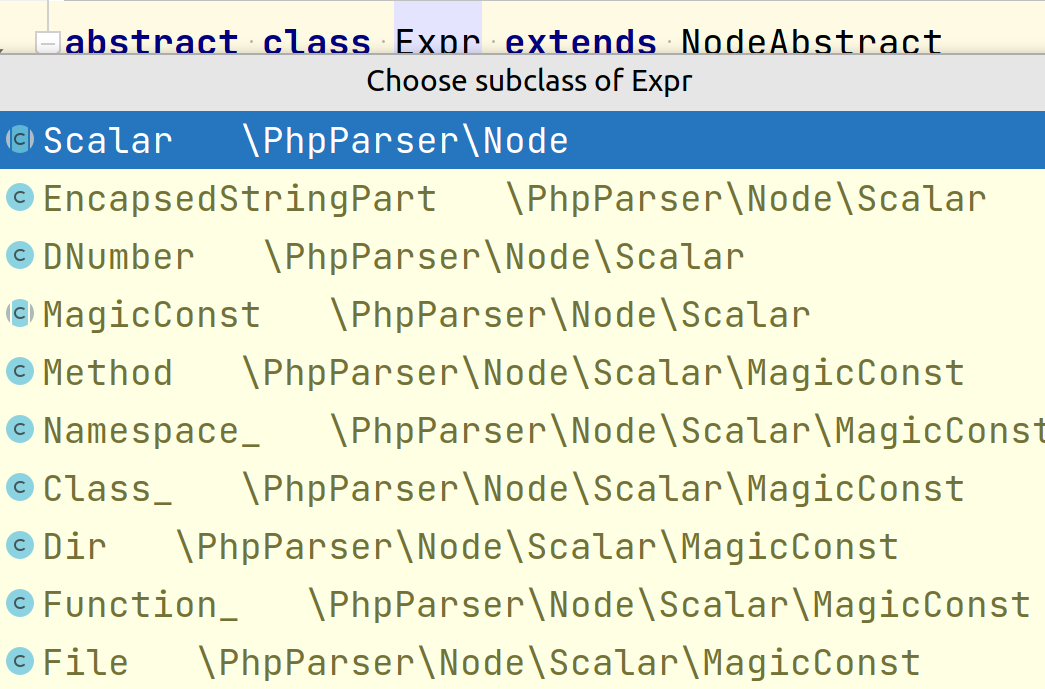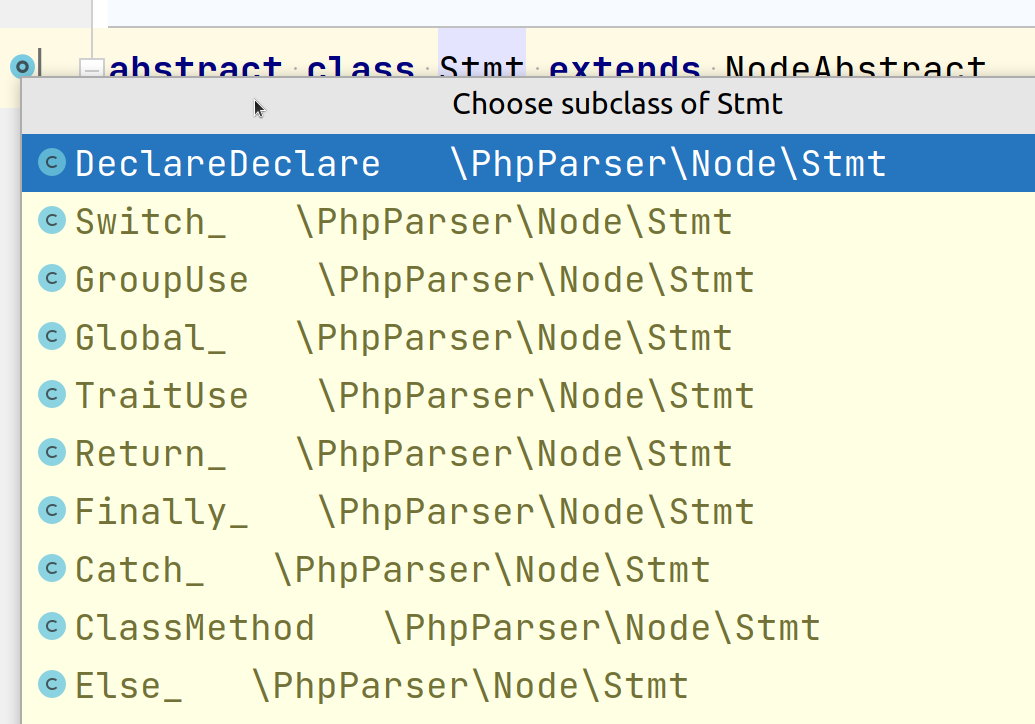# How to Replace Single Node with Two Nodes in Abstract Syntax Tree

Already over 120 people bought the Rector book that we released just a month ago. The continuously growing interest in abstract syntax technology makes me very happy.

It leads to more developers who can write their own custom Rector rules to improve their specific projects. That leads to more "how-to" questions in the Rector repository. I've decided to answer one of the frequent ones today.

Standard rules usually replace one node with another, or change it. There are three operations you can make. Let's take them one by one, starting from the simplest.

## 1. Change one Node

E.g. when here it renames one class in a constructor to another:

`````` public function __construct(
-    EntityManager \$entityManager
+    EntityManagerInterface \$entityManager
) {
}
``````

In Rector rule AST syntax, it looks like this:

``````use PhpParser\Node\Param;
use Rector\Core\Rector\AbstractRector;

final class RenameEntityManagerRector extends AbstractRector
{
/**
* @param Param \$node
*/
public function refactor(Node \$node)
{
// here we only change `type` property, everything else remains the same
\$node->type = new Name('EntityManagerInterface');

// then we return original node
return \$node;
}

// ...
}
``````

We change a node and return the changed node. Let's move to level 2.

## 2. Replacing One Node by Another

Let's say we want to upgrade to PHP 8 and use new `str_contains()` function:

``````-Nette\Utils\Strings::contains(\$content, 'letter');
+str_contains(\$content, 'letter');
``````

Here we replace `StaticCall` node with `FuncCall`:

``````use PhpParser\Node\Expr\FuncCall;use PhpParser\Node\Expr\StaticCall;
use PhpParser\Node\Name;use Rector\Core\Rector\AbstractRector;

final class ReplaceStrContainsRector extends AbstractRector
{
/**
* @param StaticCall \$node
*/
public function refactor(Node \$node)
{
// we'll use arguments from the original node
\$args = \$node->args;

// here we create a new func call node, with specific name and args
\$funcCallName = new Name('str_contains');

return new FuncCall(\$funcCallName, \$args);
}

// ...
}
``````

Are you still with me? Let's get to the most complex one.

## 3. Replacing Single Node by Two Nodes

Let's say we have an extremely complex line where a lot of operations happens at once:

``````\$result = 5 + 10;
``````

I'm a bit exaggerating here, but I believe any code should be easily readable even after 3 glasses of wine. How can we improve the code, so we understand the code even late in the night?

Let's separate one operation per line:

``````-\$result = 5 + 10;
+\$input = 10;
+\$result = 5 + \$input;
``````

One line per one operation. Now we can easily see what is going on. How would such modification look like in a Rector rule?

``````use PhpParser\Node\Expr\Assign;
use PhpParser\Node\Expr\BinaryOp\Plus;
use PhpParser\Node\Expr\Variable;
use Rector\Core\Rector\AbstractRector;

final class RenameEntityManagerRector extends AbstractRector
{
/**
* @param Assign \$node
*/
public function refactor(Node \$node)
{
/** @var Plus \$plus */
\$plus = \$node->expr;

// stands for: 5
\$leftSideOfPlus = \$plus->left;

// stands for: 10
\$rightSideOfPlus = \$plus->right;

// stands for: "\$result"
\$resultVariable = \$node->var;

// this will create "\$input = 10;"
\$inputVariable = new Variable('input');
\$firstLine = new Assign(\$inputVariable, \$rightSideOfPlus);

// this will create "\$result = 5 + \$input;"
\$secondLine = new Assign(\$resultVariable, new Plus(\$leftSideOfPlus, \$inputVariable));

// magic happens here?
}

// ...
}
``````

What should we return in the `refactor()` method to change the original line and add one extra line?

I'll give you a hint: we always returned the content we wanted to change in previous examples.

So what do we return here? Again, the content we want to change:

``````use PhpParser\Node;

// ...

public function refactor(Node \$node)
{
// ...

return [
\$firstLine,
\$secondLine
];
}
``````

Just instead of a single node, it's 2 nodes. How easy is that?

But in practice, this rule would not work. We need to respect the abstract syntax tree node traverser architecture of php-parser. Don't worry. We're 2 steps away from success. But first, you need to understand one big difference...

## Statements vs. Expressions

In php-parser, there are 2 kinds of nodes:

First extend `PhpParser\Node\Expr` classSecond extend `PhpParser\Node\Stmt` classLook at the first picture and pick one node from there... e.g., `PhpParser\Node\Scalar\MagicConst\Dir`. What is it?

``````__DIR__
``````

It's an expression that holds a value. `__DIR__` returns a `string` with an absolute path to the current directory.

Let's look at the second picture... what do we pick from there? E.g. `ClassMethod`. It's a method in a class, interface, or trait. It does not hold any value. It's part of a structure. A typical example is a constructor method:

``````public function __construct()
{
}
``````

### `Expr`

• holds a value
• can be used as part of operation (`__DIR__ . '/Fixture'`)
• can be nested in other expressions (`strlen(__DIR__ . '/Fixture') > 10`)

### `Stmt`

• is part of code
• it just exists
• is a structural element among other elements

If you're still missing the difference, check other classes that implement `Expr` or `Stmt`. It will give you a better idea.

## The Golden Rule of Replacing Nodes

Why do we even talk about this? Because we can only replace one `Stmt` by multiple `Stmt`.

Let's get back to our example:

``````-\$result = 5 + 10;
+\$input = 10;
+\$result = 5 + \$input;
``````

We had an `Assign` on the input... what is this node extending?

``````namespace PhpParser\Node\Expr;

use PhpParser\Node\Expr;

class Assign extends Expr
{
// ...
}
``````

It's an `Expr`, so we cannot replace it... we're screwed... or are we?

There is a neat trick to overcome this - every line that is not an `Stmt` is wrapped with an `Expression` node that is `Stmt`.

What does it mean?

``````// Assign (Expr)
\$result = 5 + 10

// Expressions (Stmt)
\$result = 5 + 10;
``````

Mind the missing `;` in the first line. With this trick, now we're able to update the original rule to make it work for us:

``````use PhpParser\Node\Stmt\Expression;
use PhpParser\Node\Expr\Assign;

/**
* @param Expression \$node
*/
public function refactor(Node \$node)
{
if (! \$node->expr instanceof Assign) {
return null;
}

\$assign = \$node->expr;

// ... here the change is the same

return [
new Expression(\$firstLine),
new Expression(\$secondLine)
];
}
``````

And that's it! Now we've replaced the `Assign` node with two different `Assign`s ✅ .

Would you like to learn more about Rector internals like this and how to make it work for your project? Get a Rector training where you'll learn practical tips and tricks in just 3 hours and save dozens of self-learning.

Happy coding!

Do you learn from my contents or use open-souce packages like Rector every day?
Consider supporting it on GitHub Sponsors. I'd really appreciate it!